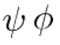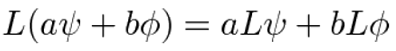# Linear Operator: Simple Definition, Examples

A linear operator is a function that maps one vector onto other vectors. They can be represented by matrices, which can be thought of as coordinate representations of linear operators (Hjortso & Wolenski, 2008). Therefore, any n x m matrix is an example of a linear operator.

An example of an operator that isn’t linear: Gα = α2.

## Formal Definition

A linear operator is usually (but not always) defined to satisfy the conditions of additivity and multiplicativity.

• Additivity: f(x + y) = f(x) + f(y) for all x and y,
• Multiplicativity: f(cx) = cf(x) for all x and all constants c.

More formally, a linear operator can be defined as a mapping A from X to Y, if:

A (αx + βy) = αAx + βAy

for all x and y in the domain of A and α, β ∈ &Copf (Hanson & Yakolev, 2002).

In quantum mechanics, a linear operator still satisfies the additivity and multiplicativity conditions, but is defined in terms of wavelengths(Branson, 2013):## Linear Operator Examples

The simplest linear operator is the identity operator, 1; It multiplies a vector by the scalar 1, leaving any vector unchanged. Another example: a scalar multiple b · 1 (usually written as just b), which multiplies a vector by the scalar b (Jordan, 2012). In fact, any multiplicative constant is also a simple linear operator.

Tensors are a type of linear operator frequently encountered in engineering and physics. In calculus, differential operators are linear operators (Branson, 2013), although they usually aren’t referred to as such in a basic calculus class. The formal rule above can be written in terms of a derivative:And an integral:## References

Hanson, G. & Yakolev, A. (2012). Operator Theory for Magnetics: an introduction. Springer.
Hjortso, M. & Wolenski, P. (2008). Linear Mathematical Models in Chemical Engineering. World Scientific Publishing Company.
Jordan, T. (2012). Linear Operators for Quantum Mechanics. Dover Publications.

CITE THIS AS:
Stephanie Glen. "Linear Operator: Simple Definition, Examples" From StatisticsHowTo.com: Elementary Statistics for the rest of us! https://www.statisticshowto.com/linear-operator/